Geometry

# Properties of Trapezoids (US) / Trapeziums (UK)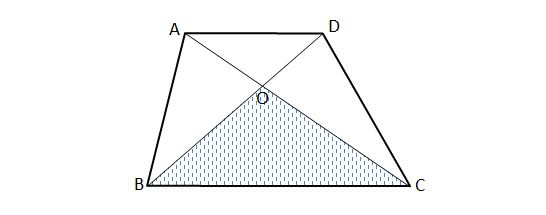In the above diagram, quadrilateral $ABCD$ is a trapezoid with $\overline{AD} \parallel \overline{BC}.$ If $\lvert{\triangle ABC}\rvert=60, \lvert{\triangle OCD}\rvert=20,$ what is the area of $\triangle OBC ?$

Note $1$: The above diagram is not drawn to scale, and $\lvert \triangle ABC \rvert$ denotes the area of $\triangle ABC.$
Note $2$: In the US a quadrilateral with one pair of parallel lines is called a "trapezoid." Elsewhere, a quadrilateral with one pair of parallel lines is called a "trapezium."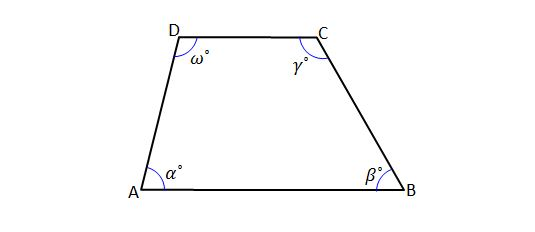In the above diagram, quadrilateral $ABCD$ is a trapezoid with $\overline{AB} \parallel \overline{CD}.$ If $\alpha^\circ={73}^\circ$ and $\gamma^\circ={135}^\circ,$ what is the value of $\omega-\beta ?$

Note: In the US a quadrilateral with one pair of parallel lines is called a "trapezoid." Elsewhere, a quadrilateral with one pair of parallel lines is called a "trapezium."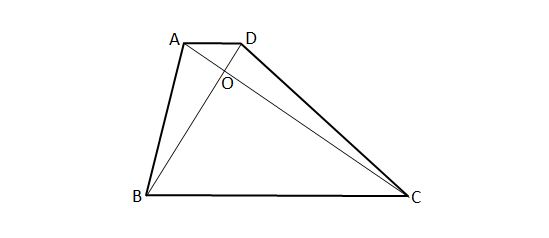In the above diagram, quadrilateral $ABCD$ is a trapezoid with $\overline{AD} \parallel \overline{BC}.$ If $\lvert{\overline{AO}}\rvert:\lvert{\overline{OC}}\rvert=1:3, \lvert{\triangle OBC}\rvert=54,$ what is the area of quadrilateral $ABCD ?$

Note $1$: The above diagram is not drawn to scale, and $\lvert{\triangle ABC}\rvert$ denotes the area of $\triangle ABC.$
Note $2$: In the US a quadrilateral with one pair of parallel lines is called a "trapezoid." Elsewhere, a quadrilateral with one pair of parallel lines is called a "trapezium."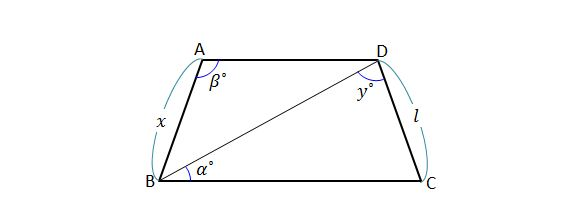In the above diagram, quadrilateral $ABCD$ is an isosceles trapezoid with $\overline{AD} \parallel \overline{BC}.$ If $l=7, \alpha^\circ={25}^\circ, \beta^\circ={115}^\circ,$ what is the value of $x+y ?$

Note $1$: The above diagram is not drawn to scale.
Note $2$: In the US a quadrilateral with one pair of parallel lines is called a "trapezoid." Elsewhere, a quadrilateral with one pair of parallel lines is called a "trapezium."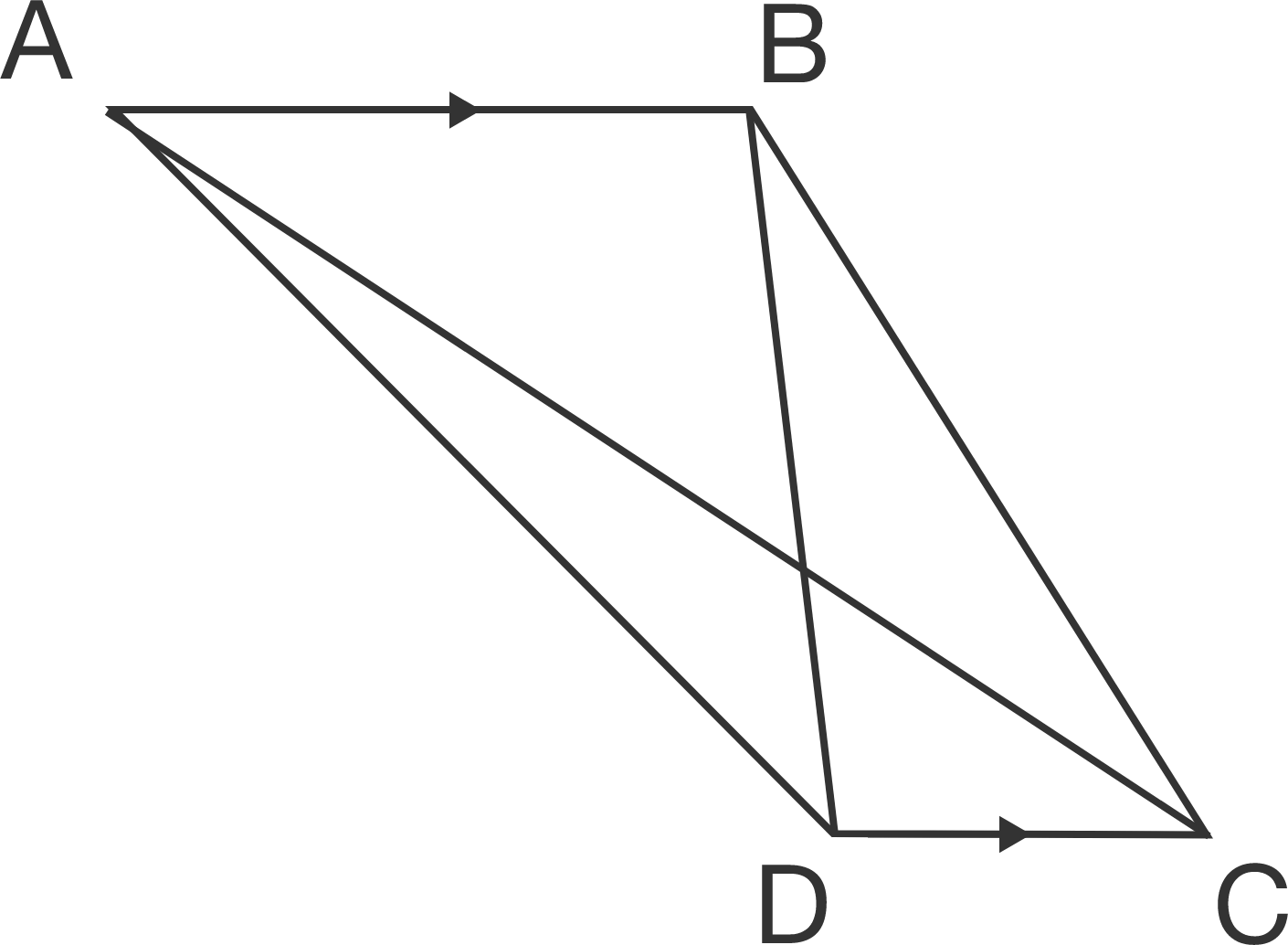Trapezium $ABCD$ has $AB$ parallel to $DC$. If $\angle DBC = 23 ^\circ$ and $\angle BCD = 59 ^\circ$, what is $\angle ABD$ (in degrees)?

×